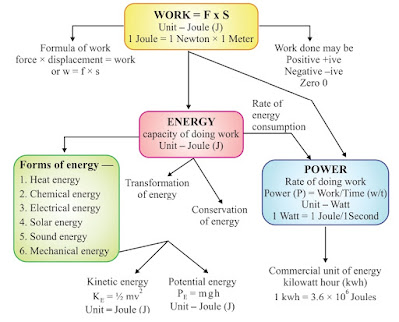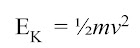## 9th Class Science Notes in English chapter 11 Work and Energy

### CBSE Revision Notes for CBSE Class 09 Science Work and Energy Work and Energy: Work done by a Force, Energy, power; Kinetic and Potential energy; Law of conservation of energy for class 09 Science. It includes all main concepts of the chapter in CBSE class 09 Science.

Class 9th Science chapter 11 Work and Energy Notes in English.

# 📚 Chapter - 11 📚👉 Work and Energy 👈## ✳️Work :-

🔹 When a force acts on an object and the object shows displacement, the force has done work on the object.

### 👉 Sign Convetions for Work Done :-

🔹 When both the force and the displacement are in the same direction, positive work is done.
▶ W = F x s

🔹 When force acts in a direction opposite to the direction of displacement, the work done is negative.
▶ W = -F x s

### 👉 Two conditions need to be satisfied for work to be done :-

🔹 A force should act on object
🔹 The object must be displaced

Unit of Work = Newton Metre or Joule

🔹 1 Joule is defined as the amount of work done by force of 1 N  when displacement is 1m

### 👉 The amount of work done depends on the following factors :-

🔹 1. Magnitude of force:- Greater the force, greater is the amount of work & vice-versa.
🔹 2. Displacement:- Greater the displacement, greater is the amount of work & vice-versa.

## ✳️Energy :-

🔹 The capacity of doing work is known as energy.

🔹 The SI unit of energy is Joule (J)

🔹 1KJ = 1000 J

### 👉 Forms of Energy :-

🔹 The various forms of energy are potential energy, kinetic energy, heat energy, chemical energy, electrical energy and light energy.

## ✳️Mechanical Energy :-

🔹 The energy possessed by a body on account of its motion or position is called Mechanical Energy.

## ✳️Kinetic Energy :-

🔹 The energy of a body due to its motion is called kinetic energy.

#### ▶ 5. A moving car

🔹 Kinetic energy is directly proportional to mass and the square of velocity.✳️Potential Energy :-

🔹 The energy of a body due to its position or change in shape is known as potential energy.

Examples are:-

#### ▶ 2. Wound up spring of a toy car

▶ 3. Bent string of bow

### 👉 Types of Potential Energy :-

🔹 On the basis of position and change in shape of object, potential energy is of two type:

1. Gravitational Potential Energy:

🔹 It is the energy possessed by a body due to it position above the ground.

2. Elastic Potential Energy:

🔹 It is the energy possessed by a body due to its change in shape.

## ✳️Transformation of Energy :-

#### ▶ The change of one form of energy to another form of energy is known as transformation of energy

🔹 1. A stone on a certain height has entire potential energy. But when it starts moving downward, potential energy of stone goes on decreasing as height goes on decreasing but its kinetic energy goes on increasing as velocity of stone goes on increasing. At the time stone reaches the ground, potential energy becomes zero and kinetic energy is maximum.

🔹 2. At hydroelectric power house, the potential energy of water is transformed into kinetic energy and then into electrical energy.

🔹 3. At thermal power house, chemical energy of coal is changed into heat energy, which is futher converted into kinetic energy and electrical energy.

🔹 4. Plants use solar energy to make chemical energy in food by the process of photosynthesis.

## ✳️Law of Conservation of Energy :-

🔹 Energy can neither be created nor destroyed, it can only be transformed from one form to another. The total energy before and after transformation remains the same.

Potential energy + Kinetic energy = Constant (Mechanical energy)

🔹 A body of mass 'm' is raised to height 'h' at A its potential energy is maximum and kinetic energy is 0.

🔹 When body falls at B, h is decreasing hence potential energy decreases and V is increasing hence kinetic energy is increasing.

🔹 When body is about to reach the ground level, h=0, V will be maximum hence kinetic energy would be at highest level.

## ✳️Power :-

🔹 The time rate of doing work is defined as power (P).

Power= work/time

### 👉 Commercial Unit of Energy :-

🔹 Commercial unit of energy is kilo watt hour (kWh)
🔹 The unit kilowatt-hour means one kilowatt of power supplied for one hour.

1 kWh = 1 kW x 1 h

= 1000 W x 60 x 60 s

= 1000 Js-1 x 3600 s

= 3.6 x 106 J

1 unit = 1 kilowatt hour = 3.6x106 J.